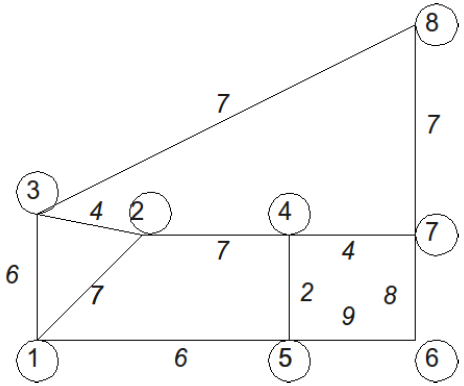## Problem S4: Animal Farm

You are running a farm which has N (1 ≤ N ≤ 100) animals. You went to the store and bought M = N pre-made pens that will house your animals. Pens satisfy the following conditions:

• pens have between 3 and 8 edges;
• an edge that is specified by two pens connects the two pens;
• an edge that is specified only once connects that pen to the outside;
• there is exactly one animal in each pen and no animals outside the pens, initially.

The animals, however, have a game they like to play called "Escape from the pen." They assign a cost to each edge of the pen, and they determine the minimum cost for all of the animals to meet in the same area by trampling over the edge of various pens. The animals may meet inside a particular pen or outside of all the pens. Also note that once an edge has been trampled, any animal may pass over it without incurring any cost.

You will be given a description of the pens, along with the placement of animals, and you are to figure out what the smallest cost is to move all the animals into the same area.

### Input

The first line of input will be the integer M, the number of pens. On the next M lines, there will be a description of each pen, with one description per line. The description is composed of three components, with each component separated by one space, as follows:

• the first component is an integer ep (3 ≤ ep), which describes the number of edges for this particular pen p;
• the second component is a sequence of ep integers describing the corners of each pen, where each integer is less than or equal to 1000;
• the third component is a sequence of ep integers describing the cost of each edge, where each integer is less than or equal to 5000.

For the corner and edge cost description, the descriptions are given in cyclical order. For example, the pen description "3 1 2 3 7 4 6" means that there are three corners, and thus, three edges, where the edge (1,2) has cost 7, the edge (2,3) has cost 4 and the edge (3,1) has cost 6. Note: at least 20% of the marks for this question have N ≤ 10 and no pen will have more than four edges in these cases.

### Output

On one line, output the minimal cost that will allow all the animals to gather in one pen or outside all of the pens.

### Sample Input

```4
3 1 2 3 7 4 6
4 1 2 4 5 7 7 2 6
4 4 7 6 5 4 8 9 2
5 3 2 4 7 8 4 7 4 7 7```

`10`

### Explanation

The diagram below explains the input data:where the circled numbers are the corners, and the numbers in italics are the edge costs. Notice that if the edges (2,3), (4,5), and (4,7) are removed, all the animals can meet in the pen which has five sides.

Point Value: 15 (partial)
Time Limit: 2.00s
Memory Limit: 16M

Languages Allowed:
C++03, PAS, C, HASK, ASM, RUBY, PYTH2, JAVA, PHP, SCM, CAML, PERL, C#, C++11, PYTH3

• (0/0)
Why is my code getting WA? I've been looking over it for the past hour and a bit, but can't see any reason why that might be. I'd really appreciate a hint of some sort. I'm sure it's probably some stupid indexing problem or something, but I'm just not seeing it -_-. Thanks!

• (1/0)
You cannot use kruskals algorithm for this problem; kruskals makes a minimum spanning forest if the graph is not connected.

• (0/0)